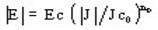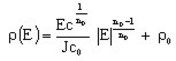# Superconductor: standard model (power law)

## Introduction

To take into account the superconducting behavior of materials, Flux offers a specific model.

## Power law

Superconducting materials have a highly nonlinear current voltage characteristic. The relationship between the electric field and the current can be described under the form of a power law:(1)The graphical form of such a law for different values of n is presented in the figure to the right.

Starting from relationship (1), we can write the resistivity for a superconductor by the following function:(2)

where:

• Ec is the critical electric field [V/m]
• Jc0 is the critical current density [A/mm2]
• n0 is an exponent
• ρ0 is an additional resistivity [Ω .m]

## Dependence on B, dependence on T

The critical current density Jc and the exponent n are the quantities that can strongly depend on the magnetic flux density B or the temperature T.

Thus the sub-models are provided to take into account these dependencies.